# Selina Concise Mathematics Class 6 ICSE Solutions Chapter 15 Decimal Fractions

## Selina Concise Mathematics Class 6 ICSE Solutions Chapter 15 Decimal Fractions

Selina Publishers Concise Mathematics Class 6 ICSE Solutions Chapter 15 Decimal Fractions

### Decimal Fractions Exercise 15A – Selina Concise Mathematics Class 6 ICSE Solutions

Question 1.
Write the number of decimal places in each of the following :
(i) 7.03
(ii) 0.509
(iii) 146.2
(iv) 0.0065
(v) 8.03207
Solution: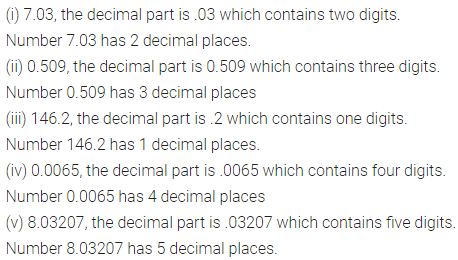Question 2.
Convert the given unlike decimal fractions into like decimal fractions:
(i) 1.36, 239.8 and 47.008
(ii) 507.0752, 8.52073 and 0.808
(iii) 459.22, 7.03093 and 0.200037
Solution: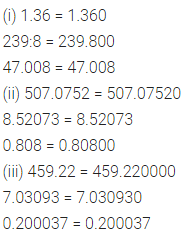Question 3.
Change each of following fractions to a decimal fraction :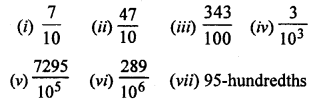Solution:Question 4.
Convert into a decimal fraction :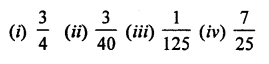Solution: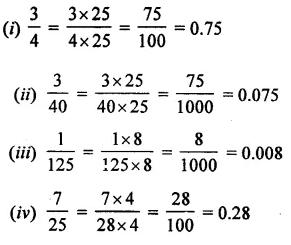Question 5.
Change the given decimals fractions to fractions in their lowest terms :
(i) 0.05
(ii) 3.95
(iii) 4.005
(iv) 0.876
(v) 50.06
(vi) 0.01075
(vii) 4.8806
Solution:### Decimal Fractions Exercise 15B – Selina Concise Mathematics Class 6 ICSE Solutions

Question 1.
(i) 0.243, 2.47 and 3.009
(ii) 0.0736, 0.6095 and 0.9107
(iii) 1.01, 257 and 0.200
(iv) 18, 200.35, 11.72 and 2.3
(v) 0.586, 0.0586 and 0.00586
Solution: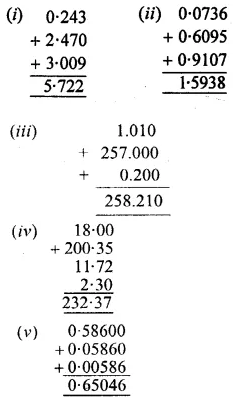Question 2.
Find the value of :
(i) 6.8 – 2.64
(ii) 2 – 1.0304
(iii) 0.1 – 0.08
(iv) 0.83 – 0.342
Solution: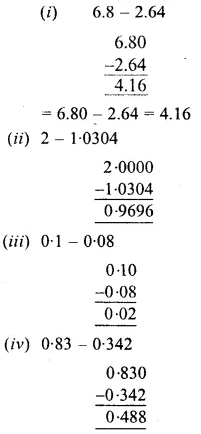Question 3.
Subtract :
(i) 0.43 from 0.97
(ii) 2.008 from 22.1058
(iii) 0.18 from 0.6
(iv) 1.002 from 17
(v) 83 from 92.05
Solution: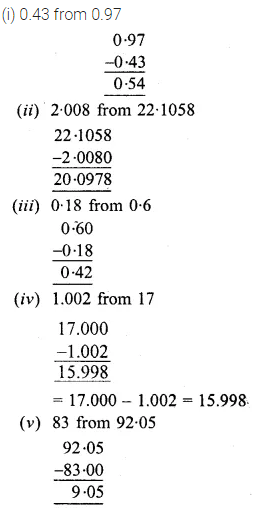Question 4.
Simplify :
(i) 3.5 – 2.43 + 0.075
(ii) 7.84 + 0.3 – 4.016
(iii) 2.987 – 1.25 – 0.54
(iv) 52.9 – 231.666 + 204
(v) 8.57 – 6.4432 – 1.70 + 0.683
Solution:Question 5.
From the sum of 75.75 and 4.9 subtract 28.465.
Solution: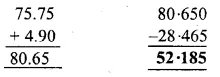Question 6.
Subtract the sum of 8.14 and 12.9 from 32.7.
Solution:Question 7.
Subtract the sum of 34.27 and 159.8 from the sum of 20.937 and 200.6.
Solution:Question 8.
From the sum of 2.43 and 4.349 subtract the sum of 0.8 and 3.15.
Solution:Question 9.
By how much does the sum of 18.0495 and 34.9644 exceed the sum of 7.6752 and 24.876?
Solution: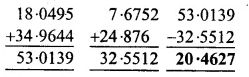Question 10.
What least number must be added to 89.376 to get 1000?
Solution: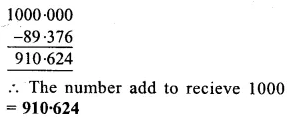### Decimal Fractions Exercise 15C – Selina Concise Mathematics Class 6 ICSE Solutions

Question 1.
Multiply :
(i) 5.6 and 8
(ii) 38.46 and 9
(iii) 0.943 and 62
(iv) 0.0453 and 35
(v) 7.5 and 2.5
(vi) 4.23 and 0.8
(vii) 83.54 and 0.07
(viii) 0.636 and 1.83
(ix) 6.4564 and 1000
(x) 0.076 and 100
Solution: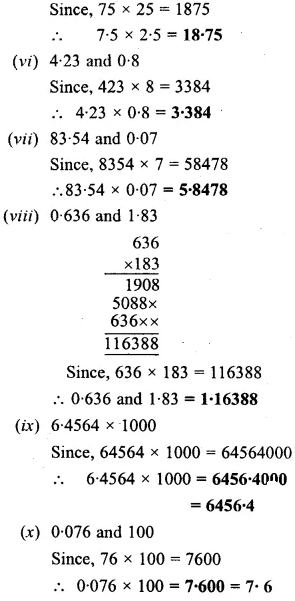Question 2.
Evaluate :
(i) 0.0008 x 26
(ii) 0.038 x 95
(iii) 1.2 x 2.4 x 3.6
(iv) 0.9 x 1.8 x 0.27
(v) 1.5 x 1.5 x 1.5
(vi) 0.025 x 0.025
(vii) 0.2 x 0.002 x 0.001
Solution: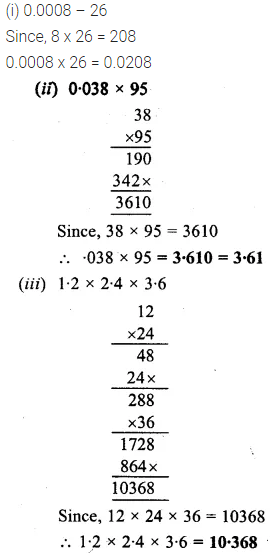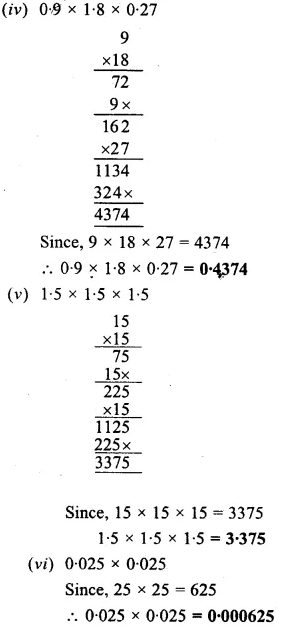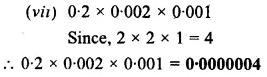Question 3.
Multiply each of the following numbers by 10, 100 and 1000 :
(i) 3.9
(ii) 2.89
(in) 0.0829
(iv) 40.3
(v) 0.3725
Solution: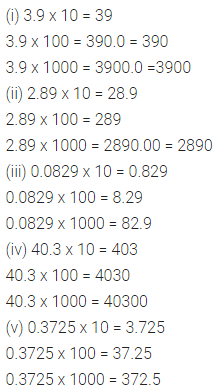Question 4.
Evaluate :
(i) 8.64 ÷ 8
(ii) 0.0072 ÷ 6
(iii) 20.64 ÷ 16
(iv) 1.602 ÷ 15
(v) 13.08 ÷ 4
(vi) 3.204 ÷ 9
(vii) 3.024 ÷ 12
(viii) 5.15 ÷ 5
(ix) 3 ÷ 5
Solution:Question 5.
Divide each of the following numbers by 10,100 and 1000 :
(i) 49.79
(ii) 0.923
(iii) 0.0704
Solution:Question 6.
Evaluate :
(i) 9.4 ÷ 0.47
(ii) 6.3 ÷ 0.09
(iii) 2.88 ÷ 1.2
(iv) 8.64 ÷ 1.6
(v) 37.188 ÷ 3.6
(vi) 16.5 ÷ 0.15
(vii) 3.2 ÷ 0.005
(viii) 3.24 ÷ 0.0016
Solution: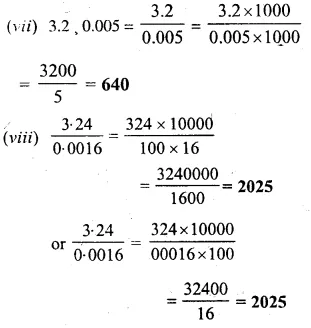Question 7.
Fill in the blanks with 10,100,1000, or 10000 etc.:
(i) 7.85 x ………….. = 78.5
(ii) 0.442 x ………. = 442
(in) 0.0924 x ………… = 9.24
(iv) 0.00187 x …………. = 18-7
(v) 2.6 x …………. = 2600
(vi) 0.08 x ………… = 80
(vii) 96.7 ÷ ………….. = 0.967
(viii) 5.2 ÷ ……………. = 0.52
(ix) 33.15 ÷ ……………….. = 0.03315
(x) 0.7 ÷ ………… = 0.007
(xi) 0.00672 x ……… = 67.2
Solution:Question 8.
Evaluate :
(i) 9.32 – 28.54 ÷ 10
(ii) 0.234 x 10 + 62.8
(iii) 3.06 x 100 – 889.4 ÷ 100
(iv) 2.86 x 7.5 + 45.4 ÷ 0.2
(v) 97.82 x 0.03 – 0.54 ÷ 0.3
Solution: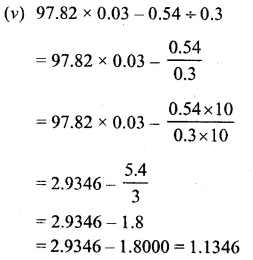### Decimal Fractions Exercise 15D – Selina Concise Mathematics Class 6 ICSE Solutions

Question 1.
Express in paise :
(i) Rs. 8.40
(ii) Rs. 0.97
(iii) Rs. 0.09
(iv) Rs. 62.35
Solution:Question 2.
Express in rupees :
(i) 55 P
(ii) 8 P
(iii) 695 P
(iv) 3279 P
Solution: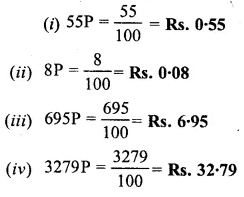Question 3.
Express in centimetre (cm) :
(i) 6 m
(ii) 8.54 m
(iii) 3.08 m
(iv) 0.87 m
(v) 0.03 m
(vi) 25.04 m
Solution:Question 4.
Express in metre (m) :
(i) 250 cm
(ii) 2328 cm
(iii) 86 cm
(iv) 4 cm
(v) 107 cm
Solution: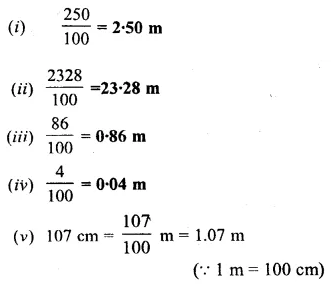Question 5.
Express in gramme (gm) :
(i) 6 kg
(ii) 5.543 kg
(iii) 0.078 kg
(iv) 3.62 kg
(v) 4.5 kg
Solution: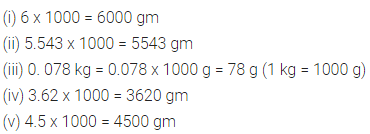Question 6.
Express in kilogramme (kg) :
(i) 7000 gm
(ii) 6839 gm
(iii) 445 gm
(iv) 8 gm
(iv) 93 gm
(vi) 13545 gm
Solution: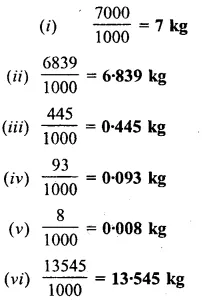Question 7.
(i) Rs. 5.37 and Rs. 12
(ii) Rs. 24.03 and 532 paise
(iii) 73 paise and Rs. 208
(iv) 8 paise and Rs. 1536
Solution: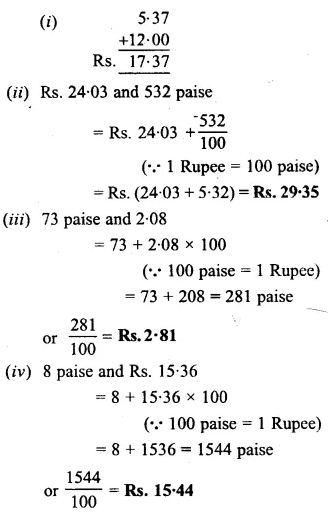Question 8.
Subtract :
(i) Rs. 35.74 from Rs. 63.22
(ii) 286 paise from Rs. 7.02
(iii) Rs. 0.55 from 121 paise
Solution: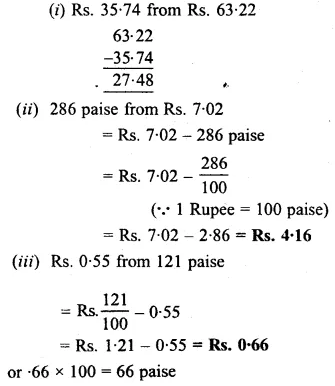Question 9.
(i) 2.4 m and 1.78 m
(ii) 848 cm and 2.9 m
(iii) 0.93 m and 64 cm
Solution:Question 10.
Subtract (giving answer in metre) :
(i) 5.03 m from 19.6 m
(ii) 428 cm from 1033 m
(iii) 0.84 m from 122 cm
Solution: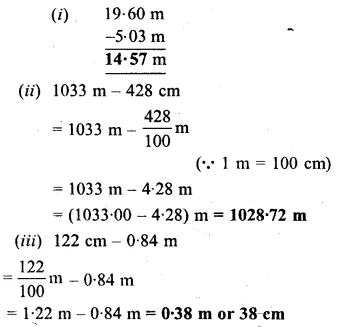Question 11.
(i) 2.06 kg and 57.864 kg
(ii) 778 gm and 1.939 kg
(iii) 0.065 kg and 4023 gm
Solution: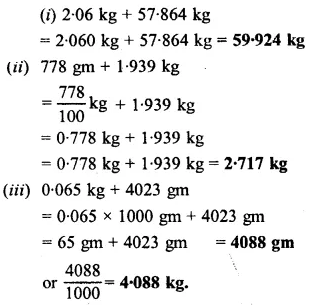Question 12.
Subtract (giving answer in kg) :
(i) 9.462 kg from 15.6 kg
(ii) 4317 gm from 23 kg
(iii) 0.798 kg from 4169 gm
Solution: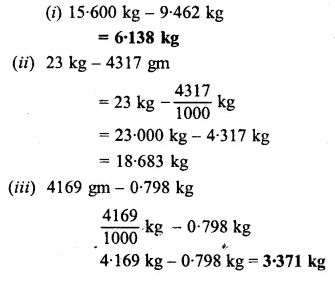### Decimal Fractions Exercise 15E – Selina Concise Mathematics Class 6 ICSE Solutions

Question 1.
The cost of a fountain pen is Rs. 13.25. Find the cost of 8 such pens.
Solution: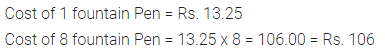Question 2.
The cost of 25 identical articles is Rs. 218.25. Find the cost of one article.
Solution: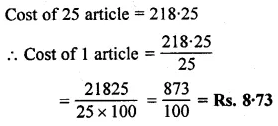Question 3.
The length of an iron rod is 10.32 m. The rod is divided into 4 pieces of equal lengths. Find the length of each piece.
Solution: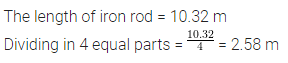Question 4.
What will be the total length of cloth required to make 5 shirts, if 2.15 m of cloth is needed for each shirt?
Solution:Question 5.
Find the distance walked by a boy in 1$$\frac { 1 }{ 2 }$$ hours, if he walks at 2.150 km every hour.
Solution: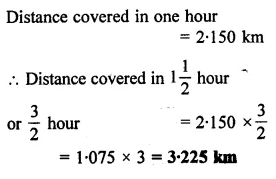Question 6.
83 note-books are sold at Rs. 15.25 each. Find the total money (in rupees) obtained by selling these note-books.
Solution: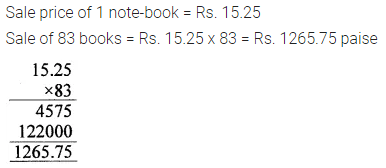Question 7.
If length of one bed-cover is 2.1 m, find the total length of 17 bed-covers.
Solution: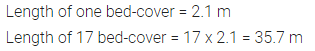Question 8.
A piece of rope is 10 m 67 cm long. Another rope is 16 m 32 cm long. By how much is the second rope longer than the first one?
Solution: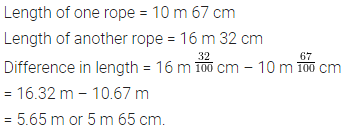Question 9.
12 cakes of soap together weigh 5 kg and 604 gm. Find the weight of
(i) One cake in both kg and gramme
(ii) 5 cakes in kg.
Solution:Question 10.
Three strings of lengths 50 m 75 cm; 68 m 58 cm and 121 m 3 cm, respectively, are joined together to get a single string of greatest length, And the length of the single string obtained.
If this single string is then divided into 12 equal pieces ; find the length of each piece.
Solution:### Decimal Fractions Revision Exercise – Selina Concise Mathematics Class 6 ICSE Solutions

Question 1.
Write th& following decimal numbers in ascending order of value
(i) 5.054, 5.250, 5.245 and 5.0543
(ii) 62.443, 62.434, 62.344 and 62.444
Solution:Question 2.
What number added to 0.805 gives 1?
Solution: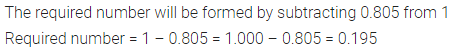Question 3.
What must be subtracted from 3 to get 2.462?
Solution: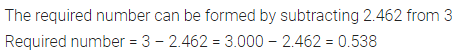Question 4.
By how much should 83.407 be decreased to get 27.78?
Solution: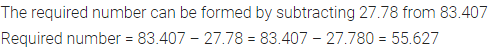Question 5.
Two articles weigh 32.674 kg and 40.038 kg respectively. Find :
(i) the total weight of both the articles.
(ii) the difference in the weights of both the articles.
Solution: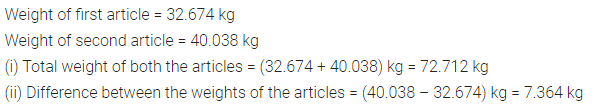Question 6.
By how much does the sum of 34.07 and 15.239 exceed the sum of 16.40 and 27.08?
Solution:Question 7.
The cost of 1 kg of fruit is Rs. 27.50. What is the cost of 3.6 kg of fruit?
Solution:Question 8.
Evaluate :
(i) 0.8 x 0.8 x 0.8
(ii) 0.8 ÷ 0.8 x 0.8
(iii) 0.8 x 0.8 ÷ 0.8
(iv) 0.8 ÷ 0.8 of 0.8
(v) 0.8 of 0.8 ÷ 0.8
Solution:Question 9.
Evaluate :
(i) 3.5 x (4.2 + 2.6)
(ii) 3.5 x 4.2 + 3.5 x 2.6
Are (i) and (ii) equal ?
Solution: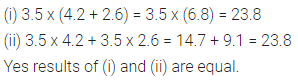Question 10.
Evaluate :
(i) (3.87 – 2.09) x 2.4
(ii) 3.87 x 2.4 – 2.09 x 2.4
Are (i) and (ii) equal ?
Solution: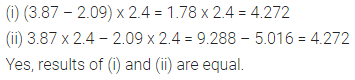Question 11.
A 4.85 m long pole is divided into 5 equal parts. Find the length of each part.
Solution: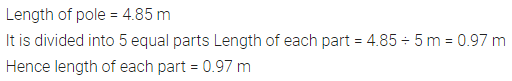Question 12.
A car can run 16.8 km consuming one litre of petrol. How many kilometres will it run on 3.7 litres of petrol ?
Solution: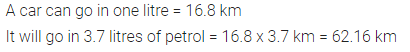Question 13.
A certain amount of money is distributed among 28 persons. If each person gets Rs. 62.45 and Rs. 5.78 is left, find the original amount of money.
Solution:Question 14.
Complete the following table :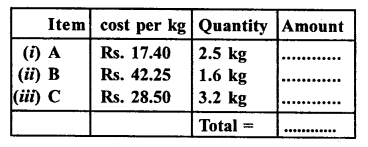Solution: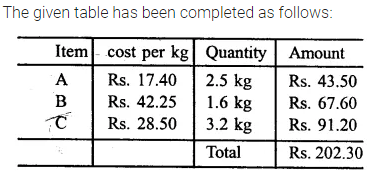Question 15.
The difference between two numbers is 47.364. If the smaller number is 31.855 ; find the bigger one.
Solution: